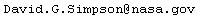## DAVID G. SIMPSON NASA Goddard Space Flight Center

 Home Publications Software Time Units Reference Educational Links Contact Me NASA GSFC

### SOFTWARE

#### Scientific Software

• Linear Regression
• e2v.f90 - Convert particle energy to velocity (Fortran-90)
• v2e.f90 - Convert particle velocity to energy (Fortran-90)
• e2t.f90 - Convert particle energy to temperature (Fortran-90)
• t2e.f90 - Convert particle temperature to energy (Fortran-90)
• v2t.f90 - Convert particle velocity to temperature (Fortran-90)
• t2v.f90 - Convert particle temperature to velocity (Fortran-90)
• cg.f90 - Calculate Clebsch-Gordan coefficients (Fortran-90)

#### Mathematical Software

• anglevec.f90 - Find separation angle between two vectors (Fortran-90)
• auxtrig.f90 - Auxiliary trigonometric functions (Fortran-90 module)
• crossprd.f90 - Vector cross product (Fortran-90 subroutine)
• cuberoot.f90 - Cube root (Fortran-90 function)
• dec2frac.f95 - Convert a decimal number to a fraction (Fortran-95)
• deviates.f90 - Random deviates for a variety of distributions (Fortran-90)
• frac.f90 - Fractional part of a number (Fortran-90 function)
• fraction.f90 - Fraction data type (Fortran-90 module)
• invhyp.f90 - Inverse hyperbolic functions (Fortran-90 module)
• m22det.f90 - Determinant of a 2×2 matrix (Fortran-90)
• m22inv.f90 - Inverse of a 2×2 matrix (Fortran-90)
• m33det.f90 - Determinant of a 3×3 matrix (Fortran-90)
• m33inv.f90 - Inverse of a 3×3 matrix (Fortran-90)
• m44det.f90 - Determinant of a 4×4 matrix (Fortran-90)
• m44inv.f90 - Inverse of a 4×4 matrix (Fortran-90)
• m55det.f90 - Determinant of a 5×5 matrix (Fortran-90)
• m55inv.f90 - Inverse of a 5×5 matrix (Fortran-90)
• m66det.f90 - Determinant of a 6×6 matrix (Fortran-90)
• m66inv.f90 - Inverse of a 6×6 matrix (Fortran-90)
• rpn.f95 - A powerful RPN calculator (Fortran-95)
• sround.f90 - Statistical rounding function (Fortran-90 function):

#### Calendar Calculations

• cal.f90 - Calendar program (similar to UNIX "cal") (Fortran-90)
• caljul.f90 - Calendar program for Julian calendar (similar to UNIX "cal") (Fortran-90)
• greg2jd.f90 - Gregorian date to Julian day (Fortran-90)
• jd2greg.f90 - Julian day to Gregorian date (Fortran-90)
• doy2greg.f90 - Day of year to Gregorian date (Fortran-90)
• greg2doy.f90 - Gregorian date to day of year (Fortran-90)
• greg2dow.f90 - Gregorian date to day of week (Fortran-90)
• leapyr.f90 - Leap year (Fortran-90)
• easter.for - Date of Easter (Fortran-77)
• Display the current date, time, day of year, and Julian day:
• jd.c - C version
• jd.f90 - Fortran-90 version

#### Computer-Related

• fix2free.f90 - Convert Fortran fixed to free format (Fortran-90)
• chcase.f90 - Convert strings to uppercase or lowercase (Fortran-90)
• toupper.f90 - Convert a file to uppercase (Fortran-90)
• tolower.f90 - Convert a file to lowercase (Fortran-90)
• dispcr.c - Display end-of-line characters (C)
• replcr.c - Convert end-of-line characters (C)
• replcrb.c - Convert end-of-line characters (batch version) (C)
• datatest.c - Display C data type sizes (C)
• byteswap.f90 - Reverse byte order of a variable (Fortran-90 module)
• timer.f90 - Compute elapsed time (Fortran-90 module)
• Display whether computer is little- or big-endian:
• Convert IEEE floating-point numbers to/from hex integers:
• ieee.c - Single precision (C)
• ieee.f90 - Single precision (Fortran-90)
• dieee.c - Double precision (C)
• dieee.f90 - Double precision (Fortran-90)

#### Miscellaneous

• scalebar.f90 - Create data for a plot color scale bar (Fortran-90)

#### Contact Information

I may be contacted at:/simpson/

Webmaster and Responsible Official: David G. Simpson
Page last updated: August 3, 2012.

Privacy Notices